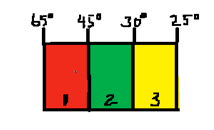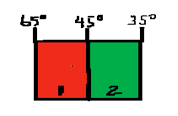# Thermal ConductivityIn physics, thermal conductivity, k, is the intensive property of a material that indicates its ability to conduct heat. It is defined as the quantity of heat, Q, transmitted in time t through a thickness L, in a direction normal to a surface of area A, due to a temperature difference ΔT, under steady state conditions and when the heat transfer is dependent only on the temperature gradient.

thermal conductivity = heat flow rate × distance / (area × temperature difference)Try the following problems, using the above equation.

The drawing shows a composite slab consisting of three materials through which heat is conducted from left to right. The materials have identical thicknesses and cross-sectional areas. Rank the materials according to their thermal conductivities, largest first.Heat is conducted through the two slabs shown in the drawing. The slabs have identical thicknesses and cross-sectional areas, but they are made from different materials. The thermal conductivity of slab 1 is k 1 = 85 J/(s · m · C ° ). What is the thermal conductivity k 2 of slab 2? Give your answer to two significant figures, e.g., 45 J/(s · m · C °).